Curl

rotation, of a vector fieldThe vector field given by the "rotational component" of this field. If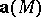is the velocity field of the particles of a moving continuous medium, the curl is equal to one-half the angular velocity of the particles. The curl is denoted by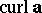(sometimes by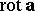). In orthogonal Cartesian coordinates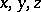the curl is defined by the expression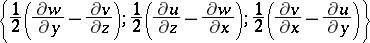where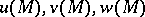are the components of.

An integral curve of the curl of a vector field is known as a vortical line. Any surface generated by a one-parameter family of vortical lines is known as a vortical surface. A very important special case of vortical surfaces are vortical tubes, formed by the vortical lines issuing from all points of some closed curve. If this curve is infinitely small, the generated vortical surface is said to be a vortical thread. Vortical surfaces are also known as vortical layers, these layers being regarded as consisting of a geometrical surface with a facing of vortical lines. When intersecting the vortical layer the velocities of the liquid particles undergo a tangential discontinuity which is proportional to the curl at the respective point.

According to the fundamental Helmholtz theorem in hydrodynamics, if the volume forces have a potential, then, during the flow of a homogeneous ideal non-compressible liquid or a barotropic gas, those particles of the medium located at a certain moment of time on a vortical line will also be on the vortical line at all successive moments of time. Thus, vortical surfaces, and in particular vortical tubes and vortical lines, are persistent in time. Any vortical tube may be characterized by some number — the strength of the tube — which is equal to the flux of the curl vector field across an arbitrary cross-section of the tube. This number is independent of the form of the cross-section, since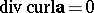. It means that the vortical tube may be either closed (a vortical ring) or may have a beginning and an end on the boundaries of the liquid. The strength of a vortical tube in an ideal liquid remains unchanged with time.

These properties of vortical tubes, discovered by H. Helmholtz, can be very simply demonstrated with the aid of W. Thomson's (Lord Kelvin's) concept of the circulationof the velocity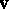along a closed contour:where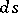is the arc element of the contouranddenotes the angle betweenand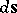. The study of the properties of velocity circulation leads to the theorem of Lagrange on the constancy of non-turbulent motion with time.

The principal task of the theory of curls is to determine the velocity field of the liquid motion along a given curl vector field. If the region occupied by the liquid is infinite in all directions and if the region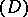occupied by the curl is bounded by a closed vortical surface, the velocity field can be found with the aid of the vector potential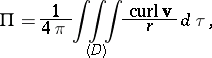from the formulaIf the task consists of determining velocities along vortical lines in a bounded space, its solution is very difficult, involving integral equations with singular kernels. For a complete solution of this problem see , .

In the important special case of plane-parallel motion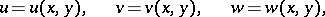two componentsof the curl are zero, while the third componentrepresents all of the curl which, in that particular case, is perpendicular to the plane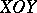. A tiny area, known as a curl point, is formed at the intersection of a curl thread with the plane. If several curl points are present in a liquid, there results a motion of the points themselves, due to the velocities they generate in the liquid. The equation of motion of curl points has the form of canonical equations of mechanics.